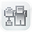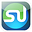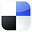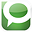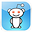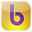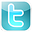Excel
STATISTICAL
Master

The t Distribution

Clear and Complete - WITH LOTS OF SOLVED PROBLEMS

# t Distribution & Small Samples

Lots of Worked-Out, Easy-To-Understand, Graduate-Level Problems  --->
( Scroll Down and Take a Look ! )

Introduction

The t Distribution is often applied to small samples (n<30) to estimate a Confidence Interval of the mean for a much larger population. It is also used to perform a Hypothesis test called a t-test which determines whether the means of two groups are statistically different from each other.

## Basic Description of t Distribution

The t Distribution curve looks very much like the bell-shaped Normal Distribution curve, except it is lower, flatter, and wider. In the real world, data often has heavier tails than the Normal Distribution describes. This is often caused by outliers. If it difficult to remove or downweigh the outliers, then the t Distribution is often used to replace the Normal Distribution.

The t Distribution is also called the Student's t Distribution. The t Distribution was first published by William Sealy Gosset in 1908. At that time W. S. Gosset was employed by the Guinness Brewery in Dublin. Due to proprietary issues, Gossett published his work under the pseudonym of "Student." Later R. A. Fisher published the t-test and referred to Gosset's distribution the "t Distribution." Since then, the distribution has been known as the Student's t distribution, or t distribution for short.

## t Distribution Probability Density Function

The Probability Density Function for the t Distribution at point t requires only 1 parameter,  ѵ, the number of Degrees of Freedom. The Degrees of Freedom, ѵ, is equal the Sample Size Minus 1 ----> ѵ = n - 1

The t Distribution depends only on ѵ, the number of Degrees of Freedom. It does not depend on the mean, µ, or the standard deviation, σ, as does the Normal Distribution. This lack of dependence on the mean or standard deviation makes the t Distribution important in practice.

t-Distribution Basic Definition

### Probability Density Function for the t Distribution = f(t,ѵ)

f(t,ѵ) = c ( 1 + t2/ѵ)-((ѵ+1)/2)

c = [ Γ( (ѵ+1)/2 ) ] / [ SQRT( ѵ * π) * Γ(ѵ/2) ]

c = [ Γ( (ѵ+1)/2 ) ] / [ SQRT( ѵ * 3.14159265) * Γ(ѵ/2) ]

Γ(x)  is the Gamma Distribution.

The above formula is difficult to work with. It is definitely better to use t Value charts to obtain the value of f(t,ѵ).  The use of t Value charts is discussed below.

## t Distribution Degrees of Freedom

The Degrees of Freedom equals the number of values of a statistic that are free to vary. In general, this is equal the number of independent sample scores that must be taken (Sample Size = n) minus the number of parameters that must be estimated during the calculation of the statistic.

The shape of a t Distribution depends upon sample size, n. An important parameter related to sample size is the Degrees of Freedom, or ѵ ("nu") ---> (also sometimes written as df )

Degrees of Freedom = ѵ = n - 1

Sample Size = n

The smaller the Degrees of Freedom, the lower and flatter is the t Distribution curve. The lowest, flattest t Distribution occurs at ѵ = 1 (sample size, n, equals 2). As the Degrees of Freedom increase, the t Distribution becomes higher and more peaked to approach the shape of the Normal Distribution curve. At approximately n = 30, the t Distribution begins to very closely resemble the Normal Distribution.

Degrees of Freedom

ѵ = Degrees of Freedom = n - 1
n = Sample Size

The number of Degrees of Freedom is the main parameter of the t Distribution.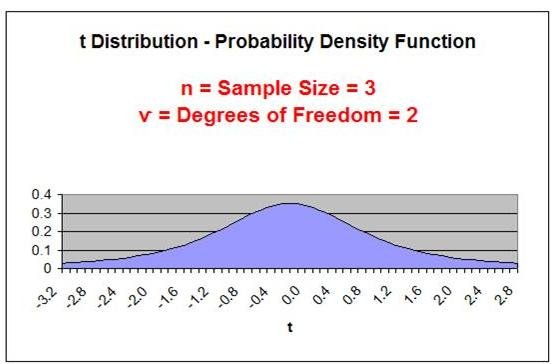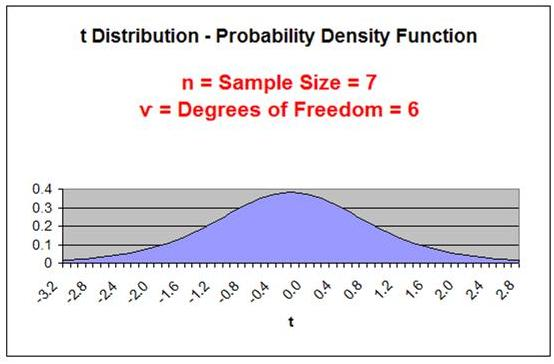As the Degrees of Freedom increase, the tails get thinner and the peak of the curve gets higher. After the Degrees of Freedom exceed 30, the t Distribution closely resembles the Normal curve, as is shown below: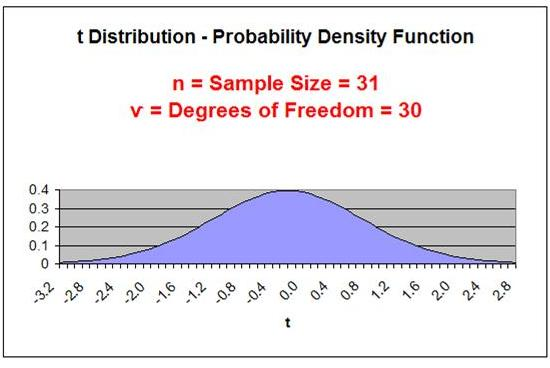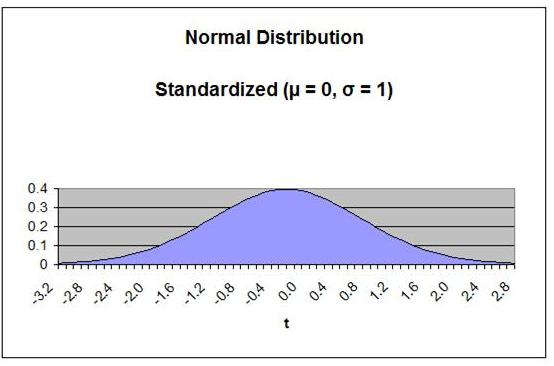One Very Important Caution

Applying the t Distribution to small samples should only be done if it can be proven that the underlying population is Normally distributed. This is not usually the case. The derivation of the t Distribution is based upon the sample being drawn from a Normally distributed population. If the t Distribution is applied to small sample data to estimate the Confidence Interval of the mean of an underlying population that is not Normally distributed, the result can be totally wrong.

Tests of normality include the Chi-Square Goodness of Fit Test. A link to this test in Excel is shown in the table of contents above.

## The Normal Distribution and Large Samples

The Normal distribution can be applied to large sample data to estimate the Confidence Interval of the mean for any population regardless of the population's distribution. Statistics' most basic rule, the Central Limit Theorem, provides the basis for this. This is explained in more detail in the course module entitled "Confidence Intervals."

If a population's underlying distribution is unknown, it is highly advisable that sample size be large (n > 30).

## Estimating Confidence Interval with the t Distribution

This course module will provide a basic explanation of the calculations of a population's Confidence Interval of the mean from small sample data using the t Distribution. A more detailed explanation of estimating a Confidence Interval  can be found in the course module entitled "Confidence Intervals."

## Levels of Confidence and Significance

Level of Significance, α ("alpha"), equals the maximum allowed percent of error. If the maximum allowed error is 5%, then α = 0.05.

Level of Confidence is selected by the user. A 95% Confidence Level is the most common. A 95% Confidence Level would correspond to a 95% Confidence Interval of the Mean. This would state that the actual population mean has a 95% probability of lying within the calculated interval. A 95% Confidence Level corresponds to a 5 Level of Significance, or α = 0.05. The Confidence Level therefore equals 1 - α.

## Population Mean vs. Sample Mean

Population Mean = µ ("mu") (This is what we are trying to estimate)

Sample Mean = xavg

## Standard Deviation and Standard Error

Standard Deviation is a measure of statistical dispersion. It's formula is the following: SQRT ( [ SUM (x - xavg)2 ] / N ). Standard Deviation equals the square root of the Variance.

Population Standard Deviation = σ ("sigma")

Sample Standard Deviation = s

Standard Error is an estimate of population Standard Deviation from data taken from a sample. If the population Standard Deviation, σ, is known, then the Sample Standard Error, sxavg, can be calculated. If only the Sample Standard Deviation, s, is known, then Sample Standard Error, sxavg, can be estimated by substituting Sample Standard Deviation, s, for Population Standard Deviation, σ, as follows:

Sample Standard Error = sxavg = σ / SQRT(n) ≈ s / SQRT(n)

σ = Population standard deviation
s = Sample standard deviation
n = sample size

## Region of Certainty vs. Region of Uncertainty

Region of Certainty is the area under the Normal curve that corresponds to the required Level of Confidence. If a 95% percent Level of Confidence is required, then the Region of Certainty will contain 95% of the area under the Normal curve. The outer boundaries of the Region of Certainty will be the outer boundaries of the Confidence Interval.

The Region of Certainty, and therefore the Confidence Interval, will be centered about the mean. Half of the Confidence Interval is on one side of the mean and half on the other side.

Region of Uncertainty is the area under the Normal curve that is outside of the Region of Certainty. Half of the Region of Uncertainty will exist in the right outer tail of the Normal curve and the other half in the left outer tail. This is similar to the concept of the "two-tailed test" that used in Hypothesis testing in further sections of this course. The concepts of one and two-tailed testing are not used when calculating Confidence Intervals. Just remember that the Region of Certainty, and therefore the Confidence Interval, are always centered about the mean on the Normal curve.

## Relationship Between Region of Certainty, Uncertainty, and Alpha

The Region of Uncertainty corresponds to α ("alpha"). If α = 0.05, then that Region of Uncertainty contains 5% of the area under the Normal curve. Half of that are (2.5%) is in each outer tail. The 95% area centered about the mean will be the Region of Certainty. The outer boundaries of this Region of Certainty will be the outer boundaries of the 95% Confidence Interval. The Level of Confidence is 95% and the Level of Significance, or maximum error allowed, is 5%.

## t Value

t Value is the number of Standard Errors from the mean to outer right boundary of the Region of Certainty (and therefore to the outer right boundary of the Confidence Interval). Standard Errors are used and not Standard Deviations because sample data is being used to calculate the Confidence Interval.

The outer right boundary of the 95% Confidence Interval, and the Region of Certainty, is 2.57 Standard Errors from the mean. The left boundary is the same distance from the mean because the Confidence Interval is centered about the mean.

## t Value Calculation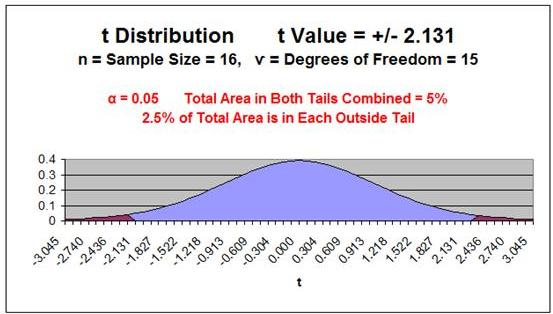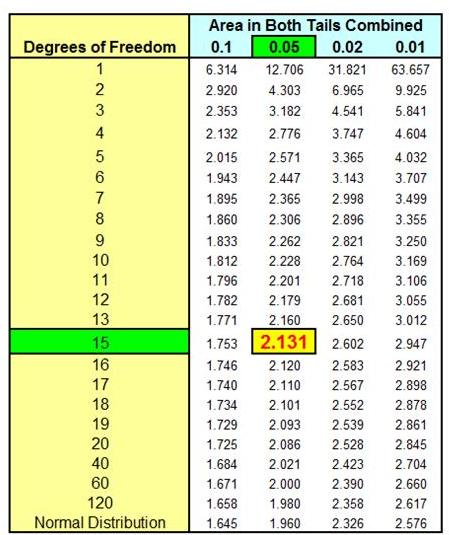## Formula for Calculating Confidence Interval  Boundaries from Sample Data Using the t Distribution

Confidence Interval Boundaries = Sample mean +/- t Value(α, ѵ) * Sample Standard Error

Confidence Interval Boundaries = xavg +/- t Value(α, ѵ) * sxavg

Problem 1

Problem 1: Calculate a Confidence Interval Based on Small Sample Data Using the t Distribution

Problem: Given the following set of 16 random test scores taken from a much larger population THAT IS NORMALLY DISTRIBUTED, calculate with 95% certainty an interval in which the population mean test score must fall. In other words, calculate the 95% Confidence Interval for the population test score mean using the t Distribution.

16 Random Test Score Samples from a Much Larger Population

 220 370 370 220 500 640 640 500 220 370 370 220 500 640 640 500

Level of Confidence = 95% = 1 - α

Level of Significance = α = 0.05

Sample Size = n = 16

Degrees of Freedom = ѵ  = n - 1 = 16 - 1 = 15

Sample Mean = xavg = 432.5

Sample Standard Deviation = SQRT ( [ SUM (x - xavg)2 ] / n ) = 160.6

Sample Standard Error = sxavg =  / SQRT(n)   s / SQRT(n)

Sample Standard Error = sxavg   s / SQRT(n) = 160.6 / SQRT(16) = 40.2

t Value
(α=0.05, ѵ = 15)  = 2.131

Width of Half the Confidence Interval  = t Value(α, ѵ) * Sxavg

Width of Half the Confidence Interval  = 2.1314 * 40.2 = 85.7

Confidence Interval Boundaries = xavg +/- t Value(α, ѵ) * Sxavg

Confidence Interval Boundaries = 432.5 +/- (2.1314)*(40.2) = 432.5 +/- 85.7

Confidence Interval Boundaries = 346.8 to 518.8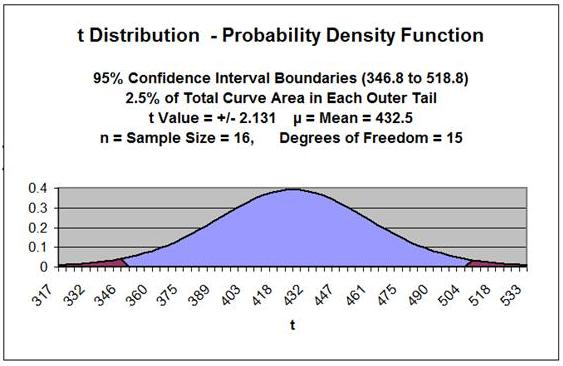## t Value CalculationThis same problem is solved in the Excel Statistical Master with only 3 Excel formulas (and not having to look up anything on a T Chart). The Excel Statistical Master teaches you everything in step-by-step frameworks. You'll never have to memorize any complicated statisical theory.

## The t Test and Hypothesis Testing

The t Test is a Hypothesis test that is used to test whether the means of two groups are statistically different from each other. Quite often the t Test is applied to small sample data. As with other applications of the t Distribution to small sample data, the underlying population must be Normally distributed. This is often not the case.

The t Test will not be covered in this module because the two modules that cover Hypothesis testing provide significant detail about the topic.

The module of this course entitled "Excel Hypothesis Tools" provides examples of three different t Tests that are Data Analysis Tools of Excel. These are:

t-Test: Paired Two Sample from Means
• t-Test: Two Sample Assuming Equal Variances (This is not a common situation)
t-Test: Two Sample Assuming Unequal Variances

Statistical Mistakes You Don't Want To Make
A Quick Normality Test Easily Done in Excel
How To Use the t Test in Excel To Find Out If Your New Marketing Is Working
The 7 Most Common Correctable Causes of Sample Data Appearing Non-Normal
Using the Excel t Test To Find Out What Your Best Sales Days Are
Nonparametric Tests - Completed Examples in Excel
Nonparametric Tests - How To Do the 4 Most Important in Excel
Nonparametric Tests - When the Marketer Should Use Them

Non-parametric tests can be used as alternatives to the t Test if large samples cannot be obtained and Normality of the underlying population cannot be proven. Some examples of applicable non-parametric tests would be the Mann-Whitney U test and the Wilcoxon test.

If You Like This, Then Share It...